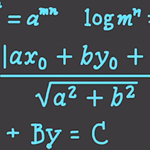# Precalculus & College AlgebraOver these next few Precalculus videos you will be 100% prepared to learn Calculus. I have found that most people think Calculus is difficult because they don’t know everything required to easily learn it. This tutorial series will solve that.

In this video I’ll teach how to : Solve Linear Equations, Find the Slope of a Line, Graph Complex Equations, Convert to Standard Form, Find Least Common Multiple, Create Equations from a Slope & Point, Find Distance between Points, Find Greatest Common Factor, Solve Inequalities, Graph Inequalities and more!